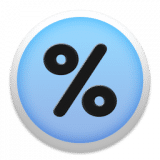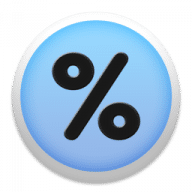PercentCalculator
1.3

0.0

PercentCalculator# PercentCalculator1.3

27 July 2015

Make percent related calculations by simply entering two values.

## Overview

PercentCalculator is a simple and effective tool which allows you to make percent related calculations instantly and effortlessly by simply entering two values.

##### Example
1. Find the percent of a number. For example, what is 37,4% of 276? Answer is 103.224007.
2. Find the percent of a part value. For example, what percentage is 42 of 300? Answer is 14%.
3. Calculate the value y% is of value x. For example, 688 if 16% of what value? Answer is 4300.
##### Features
• Copy-and-paste result
• Clean, simple, and user-friendly interface
• Menu bar app, is there when you need it

## What's new in PercentCalculator

Version 1.3:
• Icon and User Interface updated for Retina Display

#### Join over 500,000 subscribers.

Subscribe for our newsletter with best Mac offers from MacUpdate.

How would you rate PercentCalculator app?